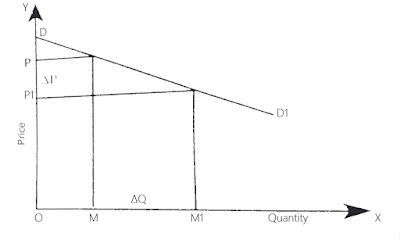### Elasticity of Demand

The term ‘elasticity’ denotes the quantity of a good to expand and contract. Hence, the change in quantity demanded due to change in price is called elasticity of demand. The economists like Courot, J. S. Mill, introduced the concept of elasticity of demand in economics. The credit is given to Dr. Alfred Marshall for the development of this concept.

The law of demand tells that the quantity demanded of a commodity varies inversely with price. But it does not tell how much quantity demanded changes with change in price. This task is accomplished by elasticity of demand. The elasticity of demand tells by how much the quantity demanded changes with change in price.
In the words of Alfred Marshall, “The elasticity (or responsiveness) of demand in a market is great or small according as the amount demanded increase much or little for a given fall in price and diminishes much or little for a given rise in price.”
According to Stonier and Hague, “Elasticity of demand is, therefore, a technical term used by the economists to describe the degree of responsiveness of the demand for the commodity to a fall in its price.”
In brief, elasticity of demand measures the rate of change in quantity demanded as a result of the change in price.

### Kinds of Elasticity of Demand

Broadly speaking, there are three main types of elasticity of demand. They are price elasticity, income elasticity and cross elasticity.

### Price Elasticity of Demand

In general, elasticity of demand means price elasticity of demand. This concept is most popular and most frequently used. Price elasticity means the responsiveness of quantity demanded to the change in price. The price elasticity of demand is defined to be the percentage change in quantity demanded resulting from 1 percent change in price. The price elasticity shows at what rate the demand changes with change in price. In the words of C. E. Ferguson, “Price elasticity is the proportionate change in quantity demanded divided by the proportionate change in price.”

The formula to find out price elasticity is,

Price elasticity = Proportionate change in quantity demanded/Proportionate change in price

Symbolically,

Ep = Δq/Δp x p/q

Where Ep = elasticity of price, P = price, Δ = small change and q = quantity. The concept of price elasticity can be illustrated by the help of a numerical example. Suppose that the original price (P1) of a commodity is \$10 per unit and quantity demanded (Q1) is 2000 units. Now suppose that when price (P2) fall to \$9, quantity demanded increases to 2500 units. The price elasticity is calculated as follows:

Ep = Δq/Δp x p/q

= 500/-1 x 10/2000 = -5/2 = -2.5

It shows that the quantity demanded increase by 2.5 percent with one percent fall in price. The minus sign shows the inverse relationship between price and quantity demanded. In general, this sign is not used, since the inverse relationship is an implied one. 2.5 is called co-efficient of elasticity of demand. The coefficient >1, =1, <1, =0 and = ∞ shows elastic, unitary elastic, inelastic, perfectly inelastic and perfectly elastic demand respectively.

### Types of Price Elasticity

The price elasticity of demand is classified into following five parts:

1. Perfectly Elastic Demand (Ep = ∞ )
The demand is said to be perfectly elastic if the quantity demanded increases in unlimited quantity with small fall in price or quantity demanded falls to zero with a small rise in price. Such situation is rarely found in real life.Perfectly Elastic Demand
In figure, demand curve DD is a horizontal straight line or parallel to the OX axis. It shows that the negligible change in price causes infinite rise or fall in quantity demanded.

2. Perfectly Inelastic Demand (Ep = 0)
If the demand remains constant whatever be the price, demand is said to be perfectly inelastic. The case of perfectly elastic demand is also rarely found in real life.Perfectly Inelastic Demand
In the figure, the demand curve DD is a vertical straight line. It shows that the demand remains constant whatever be the change in price. As for example, even after the increase in price from OP to OP1 and fall in price from OP to OP2, the quantity demanded remains OM.

3. Relatively Elastic Demand (Ep > 1)
If there is a great change in demand with a small change in price, it is called relatively (more) elastic demand. The demand for luxury goods is considered to be more elastic.Relatively Elastic Demand
In the figure, the demand curve DD is more flatter which shows that the demand is more elastic. The small fall in price from OP to OP1, has led to greater increase in demand from OM to OM1. Likewise, demand decrease more with small increase in price.

4. Relatively Inelastic Demand (Ep < 1)
If there is small change in demand with greater change in price, the demand is said to be relatively inelastic. The demand for basic goods such as salt, matches are said to be less elastic.Relatively Inelastic Demand
In the figure, demand curve DD is steeper which shows that the demand is less elastic. The greater fall in price from OP to OP1 has caused small change in demand from OM to OM1. Likewise, great increase in price leads to small fall in demand.

5. Unitary elastic demand (Ep = 1)
If the ratio of change in demand is equal to the ratio of change in price, the demand is said to be unitary elastic. This kind of elasticity is also an imaginary one.Unitary Elastic Demand
In the figure, demand curve DD is a rectangular hyperbola, which shows that the demand is unitary elastic. The fall in price from OP to OP1 has caused equal proportionate increase in demand from OM to OM1. Likewise, when price increase, the demand decreases in the same ratio.